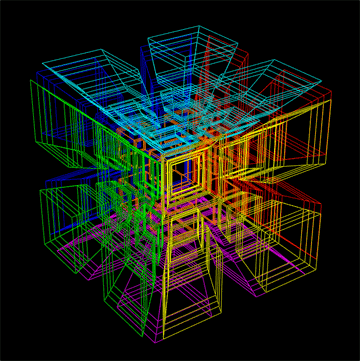# Not many, just 5 dimensions

Probability Level 2Find least possible value of $n$, such that we can always choose 2 points out of $n$ points in 5-dimensional space (wherever they may be marked), such that there's at least one more lattice point on the segment joining them.

Details and assumptions:-

• In the 5D space, every point can be represented as coordinates $(a,b,c,d,e)$, where $a,b,c,d,e,f \in \mathbb{R}$
• Lattice points are points that have integer coordinates.
×

Problem Loading...

Note Loading...

Set Loading...